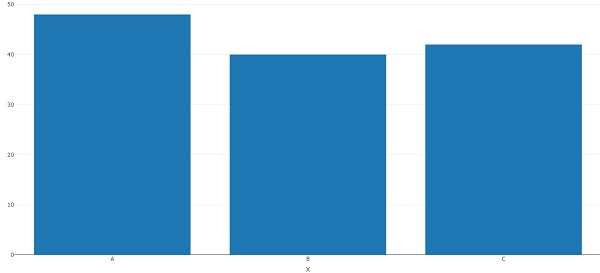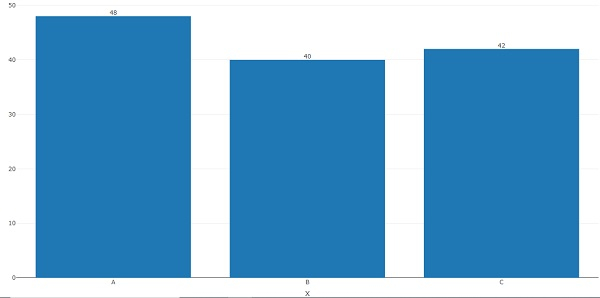# How to create plotly bar chart with values on top of bars in R?

To create plotly bar chart with values on top of bars in R, we can follow the below steps −

• First of all, create two vectors one having categories and one counts and create the bar chart using plotly.
• Then, create the same bar chart with add_text function of plotly package.

## Create the vectors and plotly bar chart

Using c function to create the vectors and plotly function with layout function to create the bar chart with axes titles −

x<-c("A","B","C")
freq<-c(48,40,42)
library(plotly)
plot_ly(x=x,y=freq,type="bar")%>%layout(xaxis=list(title="X"),yaxis=list(title="Y"))

## Output## Create the bar chart with counts on top of the bars

Using add_text function to create the bar chart with counts on top of the bars −

x<-c("A","B","C")
freq<-c(48,40,42)
library(plotly)

plot_ly(x=x,y=freq,type="bar")%>%layout(xaxis=list(title="X"),yaxis=list(title="Y"))%
>%add_text(x=x,y=freq,text=freq,textposition="top")

## Output